## How large a sample is needed if we wish to be 96% confident that our sample proportion in Exercise 9.53 will be within 0.02 of the true frac

Question

How large a sample is needed if we wish to be 96% confident that our sample proportion in Exercise 9.53 will be within 0.02 of the true fraction of the voting population?
A. A random sample of 200 voters in a town is selected, and 114 are found to support an annexation suit. Find the 96% conﬁdence interval for the fraction of the voting population favoring the suit.
B. What can we assert with 96% conﬁdence about the possible size of our error if we estimate the fraction of voters favoring the annexation suit to be 0.57?

in progress 0
6 months 2021-08-23T10:30:27+00:00 1 Answers 0 views 0

## Answers ( )

1. Complete Question

How large a sample is needed if we wish to be 96% confident that our sample proportion in Exercise 9.53 will be within 0.02 of the true fraction of the voting population?

Exercise 9.53

A. A random sample of 200 voters in a town is selected, and 114 are found to support an annexation suit. Find the 96% conﬁdence interval for the fraction of the voting population favoring the suit.

B. What can we assert with 96% conﬁdence about the possible size of our error if we estimate the fraction of voters favoring the annexation suit to be 0.57?

The First question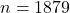A

The 96% confidence interval is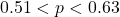B

The possible size of our error if we estimate the fraction of voters favoring the annexation suit to be 0.57 is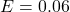Step-by-step explanation:

From the question we are told that

The margin of error is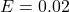The sample size is  n =  200

The number that supported the annexation suit is  k = 114

Considering the first question

Generally our sample in Exercise 9.53 is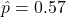From the question we are told the confidence level is  96% , hence the level of significance is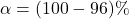=>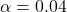Generally from the normal distribution table the critical value  of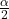is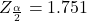Generally the sample size is mathematically represented as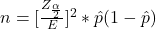=>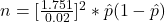=>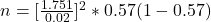=>Considering question B

Generally the margin of error is mathematically represented as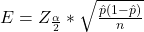=>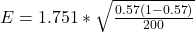=>Considering question A

Generally the sample proportion of the number that supported the annexation suit is mathematically represented as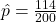=>Generally 96% confidence interval is mathematically represented as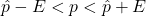=>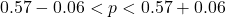=>